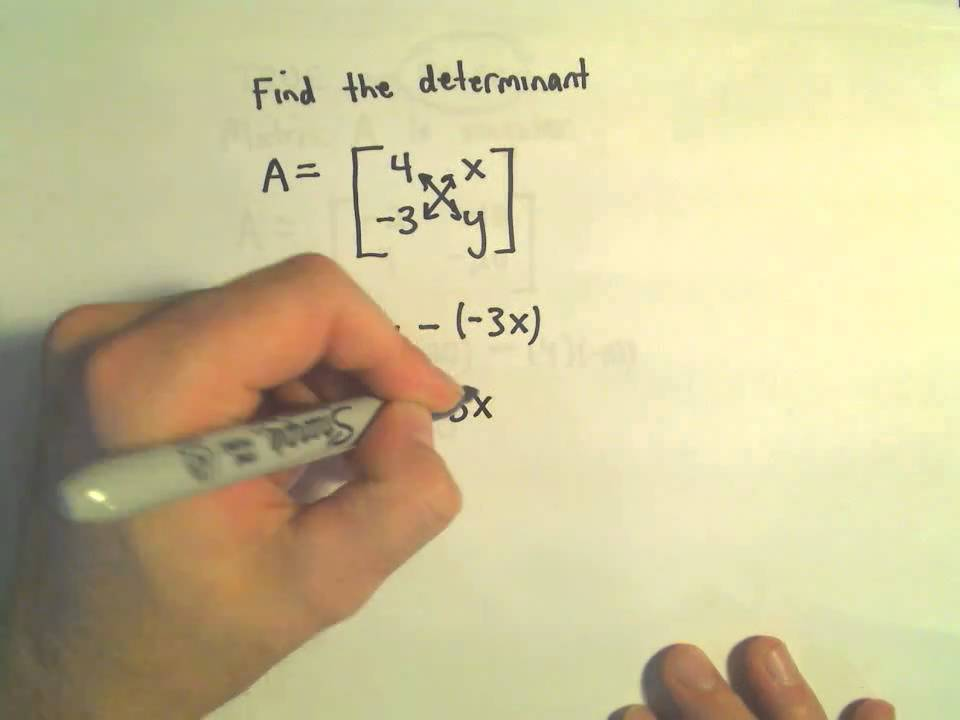# Determinants and Simplification of DeterminantsThe determinant of a square matrix is defined as the number which engraves few properties of the matrix given. The necessary condition for the matrix to be invertible is that the determinant should not be equal to 0. The word have its roots from the Latin word “determining.” It means to determine or decide on something.

Let A =The determinant is given by## Properties of Determinants Include the Following:

1] There exists a change in the sign when the rows and columns are interchanged.

2] The value of the determinant does not change even if the multiples of columns and rows are added together.

3] The scalars can be calculated from the rows and columns.

4] When a scalar “c” is multiplied to a row of a matrix, then the determinant also gets multiplied by the scalar “c”.

5] The determinant reduces to 0 when one of the rows or a column of the matrix is 0.

6] The determinant reduces to 0 when two of the rows or columns are 0.

7] The determinant can be indicated as the sum of two or more determinants when the elements of the row can be indicated as the sum of two or more elements.

8] When all the elements of a determinant above or below the main diagonal are zeros, then the determinant value = product of diagonal elements.

## Steps to Solve Determinants

To calculate the determinant of a 3 x 3 square matrix or of greater order involves a lot of time and effort as its computation is hard. The answer to the question how do you simplify determinants can be obtained here as it reduces the amount of computation. The methods for simplifying determinants involves using row or column operations to alter a few entries of the matrix to zeros.

The steps followed are given below:

1] Factor a constant from a row or column.

2] The multiples of row or column can be added to one another.

3] Two rows or columns can be interchanged.

4] The determinants can be simplified using the properties.

5] For an upper or a lower triangular matrix, determinant of matrix = product of the diagonal elements.

6] Gauss elimination can be used to reduce a determinant to a triangular form.

7] The value of a determinant consisting of two proportional rows must be 0.

8] The row operation doesn’t change the value of a determinant.

9] The row operation changes the sign of the determinant.

## Few Applications of Determinants Are as Follows.

1] Determinants are used to find whether a matrix has an inverse, and calculate the inverse using cofactors.

2] Application of Cramer’s Rule to obtain the solution of a 2 × 2  or a 3 × 3  linear system of equations.

3] To obtain an appropriate interpolating polynomial and to use it in the estimation of points.

4] The absolute value of the determinant of real vectors = the volume of the parallelepiped spanned by the vectors.

5] The determinant of a matrix is zero when the column vectors (or the row vectors) of the matrix are linearly dependent.

There exist numerous applications of determinants, a few are listed above for reference.